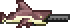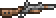# 使用时间

2020-07-10更新

≤8 超快
9-20 很快
21-25
26-30 普通
31-35
36-45 很慢
46-55 极慢
≥56 蜗牛

### 修饰使用时间

“+10% 速度”的修饰语实际上意味着“-10% 使用时间”，修饰后的值取整后得到新的使用时间。由于要取整，实际收益可能是一个不同的百分比。以下表格对比了快速发射的巨兽鲨和慢得多的火枪## 计算

$\displaystyle{ \text{total use time} = floor(round[\text{base use time}\ *\ (1-\frac{\text{weapon modifier}}{100})]\ *\ [1 / (1+\frac{\text{total attack speed modifiers}}{100})])\ +\ \text{use delay} }$（参见下文

total use time = 总使用时间，base use time = 基础使用时间，weapon modifier = 武器修饰语，total attack speed modifiers = 总攻击速度修饰语，use delay = 使用延迟

$\displaystyle{ \text{secondary use time (if not autofire)} = \text{total use time}\ *\ ceil(\frac{\text{total use time}}{\text{secondary use time as calculated with above formula}}) }$

secondary use time (if not autofire) = 次要攻击使用时间（如果不是自动开火），total use time = 总使用时间，secondary use time as calculated with above formula = 按以上公式计算得到的次要使用时间

## 备注

• “开火频率“是通过将每秒帧数（60）除以物品的使用时间计算得到的：
$\displaystyle{ \text{fire rate} = \frac{60}{\text{use time}} }$
fire rate = 开火频率，use time = 使用时间

$\displaystyle{ \text{fire rate} = \frac{60}{7} \approx 8.57 }$
fire rate = 开火频率

• 某些物品是例外，例如像蘑菇弱效治疗药水这样的回复药水，它们有个特殊的冷却周期；或者连枷回旋镖，它们必须先回来才能再次发射。
• 一些武器，诸如某些射弹近战武器，有着次要攻击方式。这些通常都具有自己的使用时间，与其标准使用时间不同。速度和攻击/近战速度的修饰语依然会影响到这些次要攻击。但是，如果此类武器没有自动开火能力且如果次要攻击的使用时间和标准使用时间不同，那么，在次要攻击的使用时间结束后，它要一直到主攻击结束后才能再次发射。
• 一些武器，比如发条式突击步枪，还有一个独立的属性叫做使用延迟（use delay）。这个属性不受速度修饰语或攻击速度/近战速度修饰语影响，作为使用时间的基础延长值。
• 物品的使用时间在游戏的源代码中由其 useAnimation 属性来表示。
• 具有自动挥舞能力的是种特殊情况。在此类剑到达挥舞动画的最后一帧时，它会跳回第一帧，因此实际上跳过了一帧。这意味着它的实际使用时间要比剑的工具提示和本 wiki 中所用的源代码中所述的值要少一。
1. 相应英文原文为rate of firefire rate，通常可翻译为射速。但由于游戏官方中文版将另一个游戏中的概念Velocity译为射速，同时这里的fire泛指一切武器（包括近战武器）的使用动作，译为发射不妥。因此，这里改用开火速率作为中文译名，以免混淆。